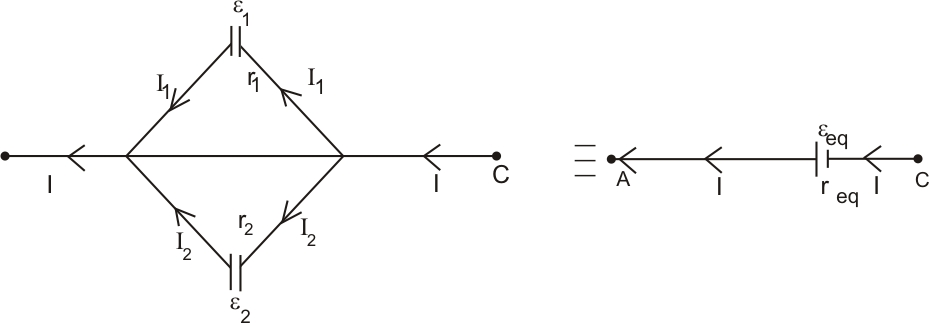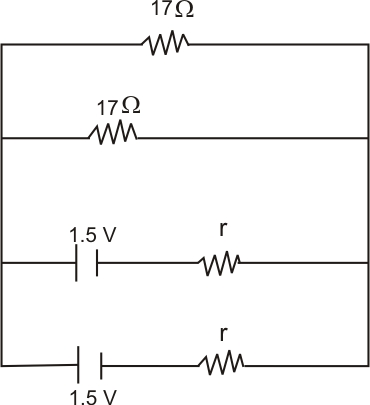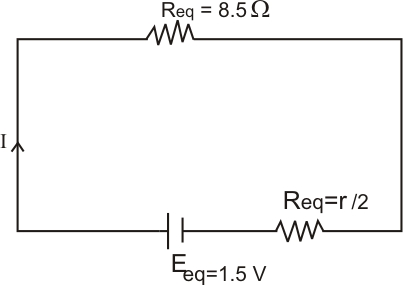# (a) Two cells of different emfs and internal resistances are connected in parallel with one another. Derive the expression for the equivalent emf and equivalent internal resistance of the combination.(b) Two identical cells of emf 1·5 V and internal resistance r are each connected in parallel providing a supply to an external circuit consisting of two resistances of each joined in parallel. A very high resistance voltmeter reads the terminal voltage of the cell to be 1·4 V. Calculate the internal resistance of each cell.

(a) Let the total amount  ______(i)and

On putting the values of  and  in equation (i) ,we get

Now, comparing with;-

Then, we get the values of  and

(b)and emf

To calculate the internal resistanceWe have,

On putting the values, we get

Now,

So,

Hence,  is the required internal resistance.

## Similar Questions

### Preparation Products

##### Knockout NEET July 2020

An exhaustive E-learning program for the complete preparation of NEET..

₹ 15999/- ₹ 6999/-
##### Rank Booster NEET 2020

This course will help student to be better prepared and study in the right direction for NEET..

₹ 9999/- ₹ 4999/-
##### Knockout JEE Main July 2020

An exhaustive E-learning program for the complete preparation of JEE Main..

₹ 12999/- ₹ 6999/-
##### Test Series NEET July 2020

Take chapter-wise, subject-wise and Complete syllabus mock tests and get in depth analysis of your test..

₹ 4999/- ₹ 2999/-